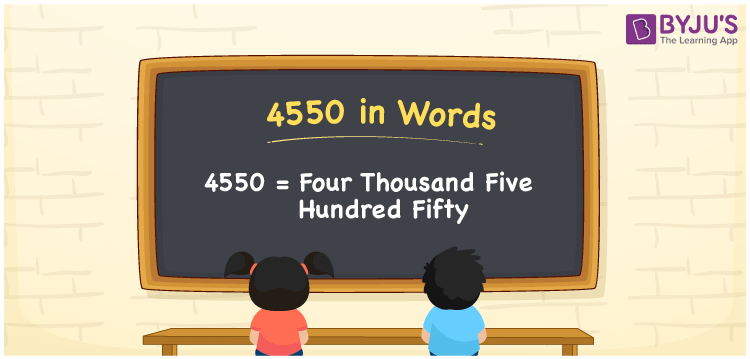# 4550 in Words

Suppose you bought a basket of fruits that cost Rs. 4550, you can say, “I bought a basket of fruits for Four thousand five hundred fifty rupees”. Thus, we can write 4550 in words as Four thousand five hundred fifty. Also, we use the word form of the cardinal number 4550 in all-around conversations. Let’s learn how to write the number 4550 in words.

 4550 in words Four thousand five hundred fifty Four thousand five hundred fifty in Numbers 4550

## 4550 in English Words

Using the English alphabet, we can express numbers in words. Thus, we can spell 4550 in English words as “Four thousand five hundred fifty”.## How to Write 4550 in Words?

We know that a place value chart is an adequate tool that can be used to convert the number 4550 into the word form. The below table shows the place values for the digits of 4550.

 Thousands Hundreds Tens Ones 4 5 5 0

Here, ones = 0, tens = 5, hundreds = 5, and thousands = 4.

Thus, we can expand the above digits as per their place values as:

4 × Thousand + 5 × Hundred + 5 × Ten + 0 × One

= 4 × 1000 + 5 × 100 + 5 × 10 + 0 × 1

= 4000 + 500 + 50

= Four thousand + Five hundred + Fifty

= Four thousand five hundred fifty

Therefore, 4550 in words = Four thousand five hundred fifty.

4550 is a natural number that precedes 4551 and succeeds 4549.

4550 in words – Four thousand five hundred fifty

Is 4550 an odd number? – No

Is 4550 an even number? – Yes

Is 4550 a prime number? – No

Is 4550 a composite number? – Yes

Is 4550 a perfect square number? – No

Is 4550 a perfect cube number? – No

## Frequently Asked Questions on 4550 in Words

Q1

### How do you say 4550 in words?

We generally spell the number 4550 in words as Four thousand five hundred fifty.
Q2

### How do you write Rs. 4550 in words on a cheque?

On a cheque, we should write Rs. 4550 in words as Four thousand five hundred fifty rupees only.
Q3

### Express the value of 4550 – 4095 in words.

4550 – 4095 = 455 Thus, the value of 4550 – 4095, i.e. 455 in words is Four hundred fifty-five.